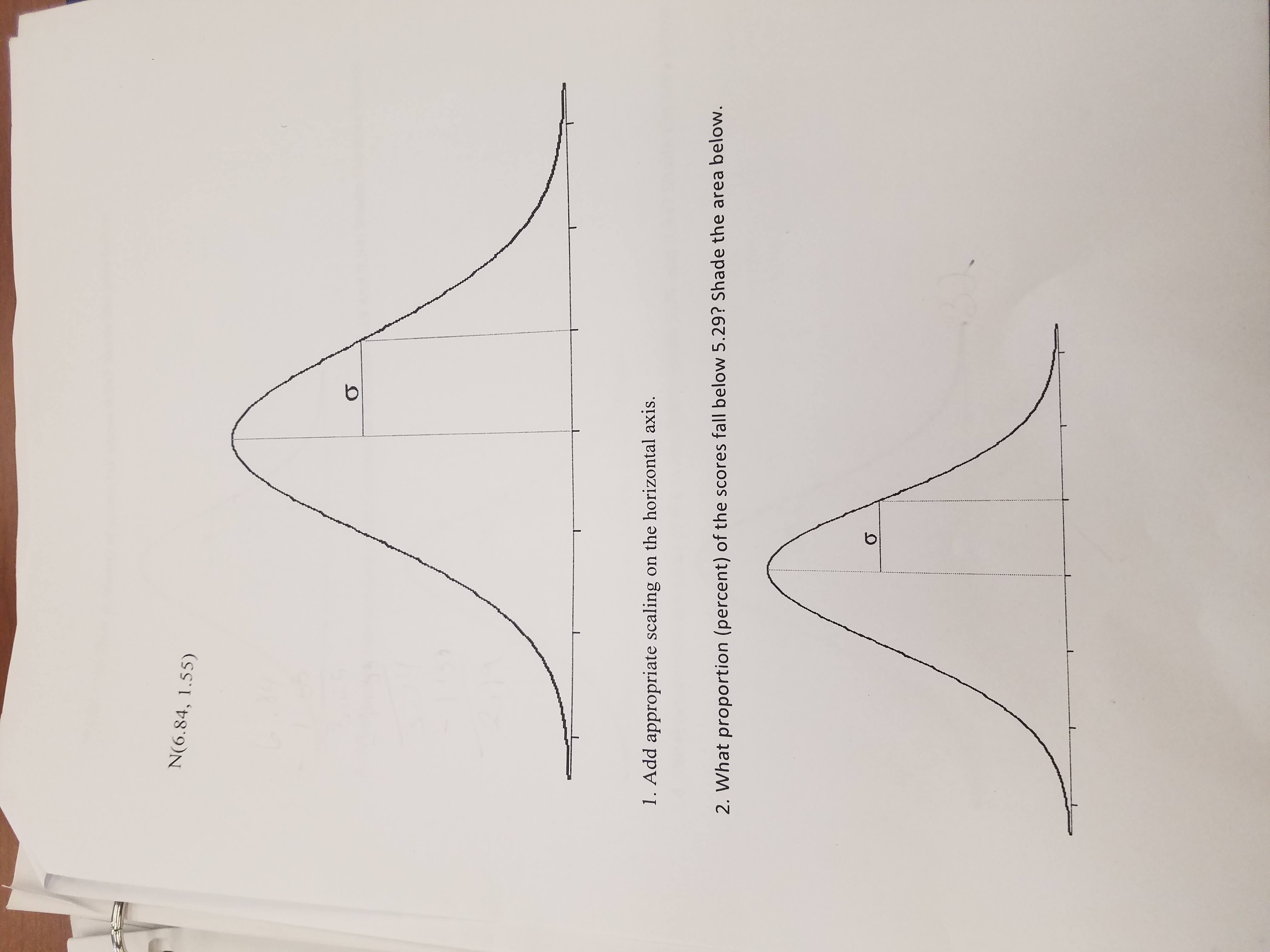N(6.84, 1.55)1. Add appropriate scaling on the horizontal axis.2. What proportion (percent) of the scores fall below 5.29? Shade the area below.

Question

I need help with this problem i was also given a table with numbers I sent it in earlierhelp_outlineImage TranscriptioncloseN(6.84, 1.55) 1. Add appropriate scaling on the horizontal axis. 2. What proportion (percent) of the scores fall below 5.29? Shade the area below. fullscreen
Step 1

A normal distribution is given with mean µ = 6.84 and standard deviation σ = 1.55.

Empirical Rule:

If a population has bell shaped distribution with mean µ and standard deviation σ ;

• Approximately 68% of the observations lie between µ - σ and µ + σ.
• Approximately 95% of the observations lie between µ - 2σ and µ + 2σ.
• Approximately all of the observations lie between µ - 3σ and µ + 3σ.
Step 2

1.

Normal distribution is a bell shaped distribution. Here using the empirical rule, the three limits for the distribution are,

Step 3

Putting the scaling in ...

Want to see the full answer?

See Solution

Want to see this answer and more?

Our solutions are written by experts, many with advanced degrees, and available 24/7

See Solution
Tagged in

Other